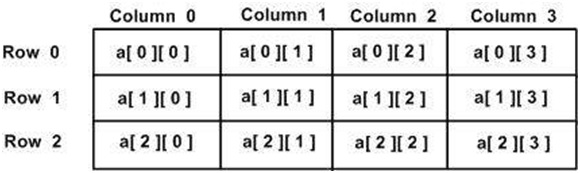# Dimensional Array in C#?

C# allows multidimensional arrays. Declare a 2-dimensional array of int as.

int [ , , ] a;

The simplest form of the multidimensional array is the 2-dimensional array. A 2-dimensional array is a list of one-dimensional arrays.

The following is a two-dimensional array with 3 rows and 4 columns.Let us now see an example to work with multi-dimensional arrays in C#.

## Example

Live Demo

using System;
namespace ArrayApplication {
class MyArray {
static void Main(string[] args) {
/* an array with 5 rows and 2 columns*/
int[,] a = new int[5, 2] {{0,0}, {1,2}, {2,4}, {3,6}, {4,8} };
int i, j;
/* output each array element's value */
for (i = 0; i < 5; i++) {
for (j = 0; j < 2; j++) {
Console.WriteLine("a[{0},{1}] = {2}", i, j, a[i,j]);
}
}
}
}
}

## Output

a[0,0] = 0
a[0,1] = 0
a[1,0] = 1
a[1,1] = 2
a[2,0] = 2
a[2,1] = 4
a[3,0] = 3
a[3,1] = 6
a[4,0] = 4
a[4,1] = 8